# Mechanical Equivalent of Heat: What is it?

## What is the Mechanical Equivalent of Heat?

There is a simple relation between mechanical work done on a system and heat generated in it. James Prescott Joule first experimentally found that the heat produced in a system is directly proportional to the mechanical work done on it. He also calculated the constant of proportionality through a unique experiment, which we will also describe in this article. The constant is popularly known as Mechanical Equivalent of Heat. After the name of James Prescott Joule, the constant is also often known as Joule’s Mechanical Equivalent of Heat or simply Joule’s Constant. We denote it with the capital English letter J.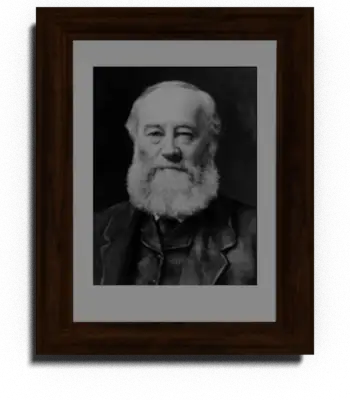If W is the work done on a system and Q is the quantity of heat produced due to this work, then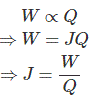The expression of J says that, the mechanical equivalent of heat is the number of units of work, that must be done on a system to produce a single unit of heat.

## Joule’s Experiment (How He Calculated Joule’s Constant)

To figure out Joule’s constant (i.e. the amount of mechanical work necessary to raise the temperature of a given mass of water by 1 degree Celsius), Joule conducted an experiment. Joule’s experiment oringinally consisted of a receptacle filled with water and a mechanism with spinning plates.

The kinetic energy of the plates is transformed into heat because the force of gravity performs work on the weight falling a distance. This gave experimental confirmation of the equivalence between heat and work, now defined to be precisely 1 calorie for every 4.184 joules and called a “thermochemical calorie”.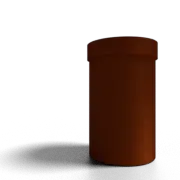For demonstrating his experiment, we will first take a cylindrical calorimeter made of copper.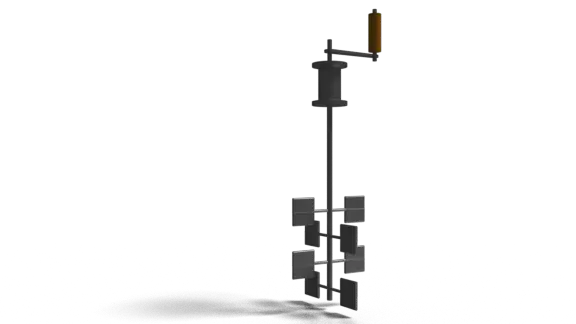We will use a vane – paddle system as shown above.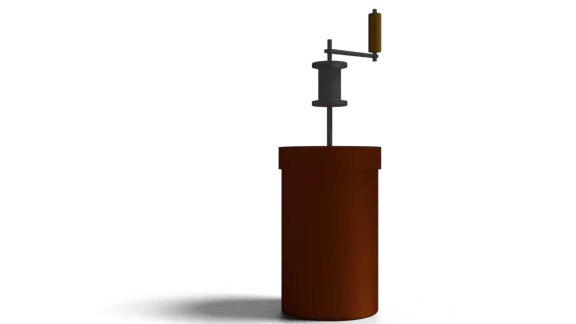Now, we will fill the calorimeter with a definite quantity of water. We will now attach the paddle – vane system to the water filled calorimeter with a watertight top cover.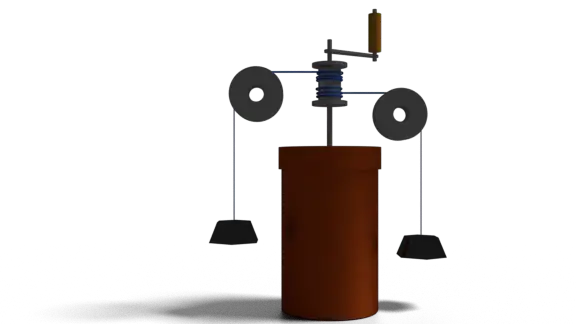Now, we will attached two weights of known and equal mass as shown in the figure above, with the help of pulleys. When we rotate the handle of the system in either direction, both weights either come up or go down vertically depending upon the direction of rotation.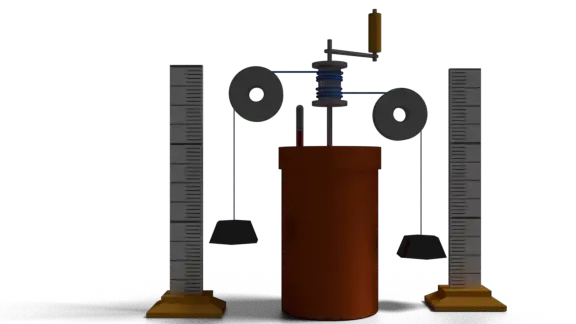We attach two vertical scales to measure the vertical; movements of the weights. We also fit one thermometer on the top cover of the system to see the temperature rise of the water.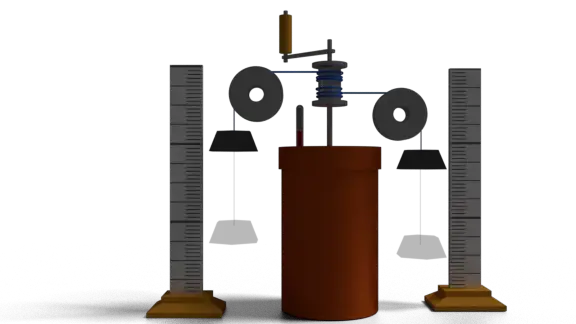Now we will lift the weights rotating the handle. After lifting the weights by height h we allow these to fall freely to its previous positions. When the weights fall, the potential energy stored in the system during lifting of the weights, is released as kinetic energy which causes rotation of the vans in the water.

This work done in the system, will generate heat in the water and it causes rise of temperature of the water. After the weights come down to their rest position, again, we will lift the weight for the same height h and allow these to come down freely. We continue, doing this until there is a measurable temperature difference indicated on the thermometer fitted in the system.

Now, we can measure the work done by multiplying total weight with the height of weights movement, number of times the weights movements repeated

Let us consider that both of the weights have the same mass m. So, the total mass of the weights is 2m. So, the work done due to the fall of weights vertically for h meters is 2mgh. Now say, total n repetitions of weight movements done before raising the temperature of the water to its measured value. Hence, total work done will beHere, all n, m, g, and h are known, so, total work done can easily be calculated.

Let us now consider, M is the mass of the water in the calorimeter. W’ is the water equivalent of the calorimeter.
So, total heat produced due to θ temperature rise of the water is,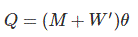Q = (M + W’)θ.
Now, mechanical equivalent of heat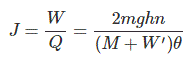After, this experiment, by putting all known values of m, g, h, n, M, W’ and θ, we get,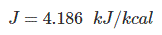Here, in this experiment, the potential energy of the falling mass is converted into the kinetic energy and finally to the heat energy.

Want To Learn Faster? 🎓
Get electrical articles delivered to your inbox every week.
No credit card required—it’s 100% free.